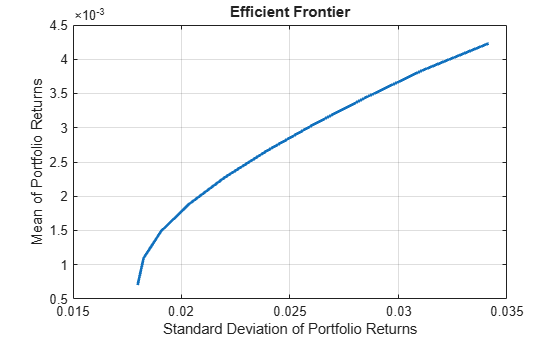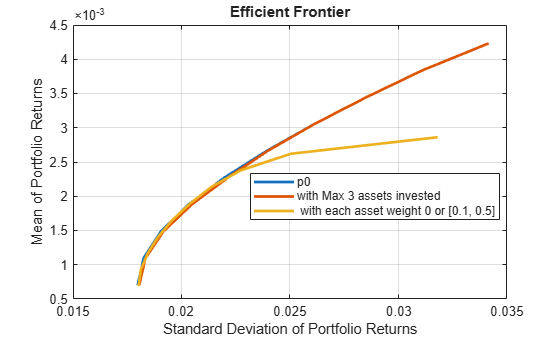plotFrontier

Plot efficient frontier

Description

example

[prsk,pret] = plotFrontier(obj) estimates the efficient frontier with default number of 10 portfolios on the frontier, and plots the corresponding efficient frontier for Portfolio, PortfolioCVaR, or PortfolioMAD objects. For details on the respective workflows when using these different objects, see Portfolio Object Workflow, PortfolioCVaR Object Workflow, and PortfolioMAD Object Workflow.

example

[prsk,pret] = plotFrontier(obj,NumPorts) estimates the efficient frontier with a specified number portfolios on the frontier, and plots the corresponding efficient frontier. The number of portfolios is defined by NumPorts.

example

[prsk,pret] = plotFrontier(obj,PortWeights) estimates efficient portfolio risks and returns with PortWeights, and plots the efficient frontier with those portfolios. This syntax assumes that you provide valid efficient portfolio weights as input. PortWeights is a NumAsset-by-NumPorts matrix.

example

[prsk,pret] = plotFrontier(obj,PortRisk,PortReturn) plots the efficient frontier with the given risks and returns. This syntax assumes that you provide valid inputs for efficient portfolio risks and returns. PortRisk and PortReturn are vectors with the same size.

Note

plotFrontier handles multiple input formats as described above. Given an asset universe with NumAssets assets and an efficient frontier with NumPorts portfolios, remember that portfolio weights are NumAsset-by-NumPorts matrices and that portfolio risks and returns are NumPorts column vectors.

Examples

collapse all

Given a portfolio p, plot the efficient frontier.

p = Portfolio('AssetList',Assets(1:12));
p = estimateAssetMoments(p, Data(:,1:12),'missingdata',true);
p = setDefaultConstraints(p);
plotFrontier(p);Create a Portfolio object for 12 stocks based on CAPMuniverse.mat.

p0 = Portfolio('AssetList',Assets(1:12));
p0 = estimateAssetMoments(p0, Data(:,1:12),'missingdata',true);
p0 = setDefaultConstraints(p0);

Use setMinMaxNumAssets to define a maximum number of 3 assets.

pWithMaxNumAssets = setMinMaxNumAssets(p0, [], 3);

Use setBounds to define a lower and upper bound and a BoundType of 'Conditional'.

pWithConditionalBound = setBounds(p0, 0.1, 0.5,'BoundType', 'Conditional');

Use plotFrontier to compare the different portfolio objects.

figure;
plotFrontier(p0); hold on;
plotFrontier(pWithMaxNumAssets); hold on;
plotFrontier(pWithConditionalBound); hold off;
legend('p0', 'with Max 3 assets invested', ' with each asset weight 0 or [0.1, 0.5]', 'location', 'best');Define a target return and use estimateFrontierByReturn to compare the three portfolio objects.

targetRetn = 2.0e-3;
pwgt0 = estimateFrontierByReturn(p0, targetRetn);
pwgtWithMaxNumAssets = estimateFrontierByReturn(pWithMaxNumAssets, targetRetn);
pwgtConditionalBound = estimateFrontierByReturn(pWithConditionalBound, targetRetn);

The following table shows the final allocation for specified target return among the three portfolio objects. You can see that the small positions in 'AAPL'and 'HPQ' are avoided in pwgtConditionalBound, and only three assets are invested in pwgtWithMaxNumAssets.

result = table(p0.AssetList',pwgt0,pwgtWithMaxNumAssets,pwgtConditionalBound)
result=12×4 table
Var1       pwgt0      pwgtWithMaxNumAssets    pwgtConditionalBound
________    ________    ____________________    ____________________

{'AAPL'}    0.076791                0                     0.1
{'AMZN'}           0                0                       0
{'CSCO'}           0                0                       0
{'DELL'}           0                0                       0
{'EBAY'}           0                0                       0
{'GOOG'}     0.44841          0.47297                 0.44255
{'HPQ' }    0.022406                0                       0
{'IBM' }     0.31139          0.34762                 0.31592
{'INTC'}           0                0                       0
{'MSFT'}     0.14101          0.17941                 0.14153
{'ORCL'}           0                0                       0
{'YHOO'}           0                0                       0

Given a PortfolioCVaR p, plot the efficient frontier.

m = [ 0.05; 0.1; 0.12; 0.18 ];
C = [ 0.0064 0.00408 0.00192 0;
0.00408 0.0289 0.0204 0.0119;
0.00192 0.0204 0.0576 0.0336;
0 0.0119 0.0336 0.1225 ];
m = m/12;
C = C/12;

AssetScenarios = mvnrnd(m, C, 20000);

p = PortfolioCVaR;
p = setScenarios(p, AssetScenarios);
p = setDefaultConstraints(p);
p = setProbabilityLevel(p, 0.95);

plotFrontier(p);Given a PortfolioMAD p, plot the efficient frontier.

m = [ 0.05; 0.1; 0.12; 0.18 ];
C = [ 0.0064 0.00408 0.00192 0;
0.00408 0.0289 0.0204 0.0119;
0.00192 0.0204 0.0576 0.0336;
0 0.0119 0.0336 0.1225 ];
m = m/12;
C = C/12;

AssetScenarios = mvnrnd(m, C, 20000);

p = setScenarios(p, AssetScenarios);
p = setDefaultConstraints(p);

plotFrontier(p);Input Arguments

collapse all

Object for portfolio, specified using Portfolio, PortfolioCVaR, or PortfolioMAD object. For more information on creating a portfolio object, see

Data Types: object

Number of points to obtain on the efficient frontier, specified as a scalar integer.

Note

If no value is specified for NumPorts, the default value is obtained from the hidden property defaultNumPorts (default value is 10). If NumPorts = 1, this function returns the portfolio specified by the hidden property defaultFrontierLimit (current default value is 'min').

Data Types: double

Standard deviations of portfolio returns for each portfolio, specified as a vector.

Note

PortRisk and PortReturn must be vectors with the same size.

Data Types: double

Means of portfolio returns for each portfolio, specified as a vector.

Note

PortRisk and PortReturn must be vectors with the same size.

Data Types: double

Optimal portfolios on the efficient frontier, specified as a NumAsset-by-NumPorts matrix.

Data Types: double

Output Arguments

collapse all

Estimated efficient portfolio risks (standard deviation of returns, returned as a vector for a Portfolio, PortfolioCVaR, or PortfolioMAD input object (obj).

Note

• If the portfolio object has a name in the Name property, the name is displayed as the title of the plot. Otherwise, the plot is labeled “Efficient Frontier.”

• If the portfolio object has an initial portfolio in the InitPort property, the initial portfolio is plotted and labeled.

• If portfolio risks and returns are inputs, make sure that risks come first in the calling sequence. In addition, if portfolio risks and returns are not sorted in ascending order, this method performs the sort. On output, the sorted moments are returned.

Estimated efficient portfolio returns, returned as a vector for a Portfolio, PortfolioCVaR, or PortfolioMAD input object (obj).

Note

• If the portfolio object has a name in the Name property, the name is displayed as the title of the plot. Otherwise, the plot is labeled “Efficient Frontier.”

• If the portfolio object has an initial portfolio in the InitPort property, the initial portfolio is plotted and labeled.

• If portfolio risks and returns are inputs, make sure that risks come first in the calling sequence. In addition, if portfolio risks and returns are not sorted in ascending order, this method performs the sort. On output, the sorted moments are returned.

Tips

You can also use dot notation to plot the efficient frontier.

[prsk, pret] = obj.plotFrontier;

Introduced in R2011a